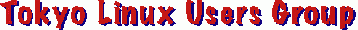Mailing List Archive

# =?UTF-8?Q?Re:_[Lingo]_Re:_Archives_should_now_ha?= =?UTF-8?Q?ndle_=E6=97=A5=E6=9C=AC=E8=AA=9E=E3=80=81English, _=D0=B8?= =?UTF-8?Q?_=D0=91=D1=8C=D0=BB=D0=B3=D0=B0=D1=80=D1=81=D0=BA=D0=B8?= =?UTF-8?Q?_/_=D0=A0=D1=83=D1=81=D1=81=D0=BA=D0=B8=D0=B9?=

```Josh Glover writes:

> Are these actually in Unicode? I know I've seen Sindarin and Quenya
> fonts, as well as Dwarvish runes, Black Speech, etc. back in the day,
> but I think they just mapped to ASCII.

Actually, they were most likely GR in ISO 8859.

AFAIK the Unicode Consortium has not added any of these so far because
they do not have a national or international standard to back them up.
Awf'ly parochial of them, to not accept a GSO (Galactic Standards
Organization) certification.

What you can do is map them into private space.  I'm fairly sure
that's how it's done in the Linux kernel.

```

Home | Main Index | Thread Index